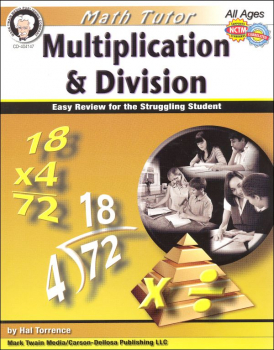# Math Tutor: Multiplication & Division

# BM-049199

Bargain Price: \$6.01
Retail: \$11.99
Save: 49.87% (\$5.98)
No more Bargain Versions available to purchase.Item #: BM-049199 9781580375764 3-6

Category Description for Math Tutor:

Need a bit of extra practice with some of your math skills - let the Math Tutor help. This series provides instruction, practice, and assessment in correlation with state, national, and provincial standards. The books in this series are all formatted similarly which makes them easy to use for independent practice in specific skill areas. Each book is 78 pages, reproducible for classroom or family use, and arranged in a systematic way where skills build on previous skills. Each section begins with "Absorb" in which the concept or skill is introduced. Key terms are highlighted in this section for easy identification. The second part of each section is "Apply" in which the students practice the new skill or concept, and "Extra Practice" is exactly that - extra practice. 2 or 3 'section reviews' are found strategically placed in which students are quizzed over the skills covered to that point, and a 'final review' is found at the end of the book which covers all skills presented in the book. Step-by-step instruction provides students with a refresher on specific topics, extra practice along with regular curriculum, or just review before a big test.

Multiplication & Division explains the functions of multiplication and division, multiplying and dividing by 1, 2, and 3 digit numbers, multiples of 10, remainders, multiplying and dividing with decimals (1, 2, or 3 places), and estimating results. Fractions & Decimals explain fractions, add/subtract/multiply/divide fractions and mixed numbers, improper fractions, ordering fractions, converting fractions to decimals, and decimals to fractions. Pre-algebra concepts include factoring, fractions, positive & negative numbers, order of operations, square and square roots, exponents, variables, problem solving, perimeter, area, volume, circles, number properties, and data analysis. Algebra concepts include factoring, fractions, positive and negative numbers, order of operations, exponents, variables, problem solving, equations, and polynomials.

Primary Subject
Mathematics
3
6
ISBN
9781580375764
EAN
044222210175
Format
Softcover Book
Brand Name
Carson-Dellosa
Weight
0.45 (lbs.)
Dimensions
10.75" x 8.5" x 0.25"